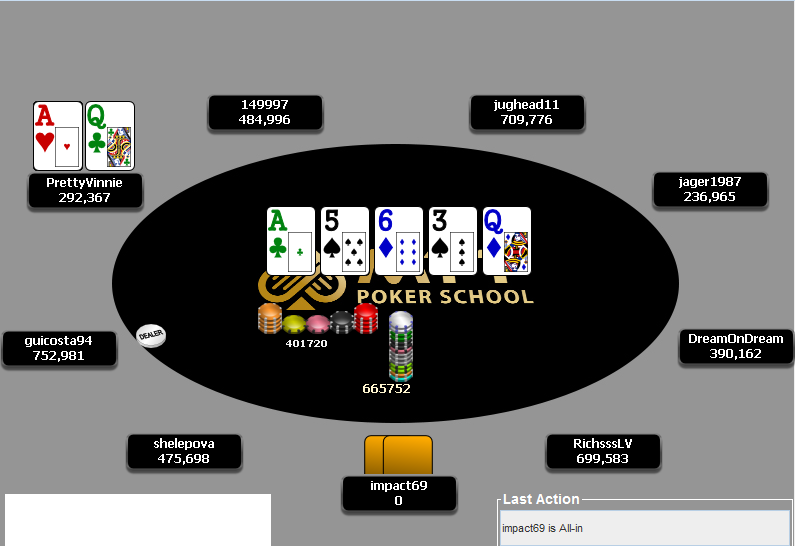# Tough Spot On The Final Table Of The Big \$55

This week I'd like to give a big shout out to PrettyVinnie who agreed for me to discuss this hand from the final table of The Big \$55 on PokerStars. As you can see from the picture he's facing a decision for all of of his chips with a seemingly very strong hand. However, there are a few things to think about before we make a decision.The hand starts off with a raise from jughead11, followed by a call from impact69 and PrettyVinnie makes the call from the Small Blind. I think Vinnie could make a case for 3-betting this hand, but he does run the risk of being put in an ICM coffin if someone chooses to 4-bet shove since he would have to fold.

On the flop jughead11 checks and impact69 bets. Now the purpose of this article is to show you the difference between Chip EV and \$ EV and how this impacts (no pun intended) your decision on the river here, which is why I'm going to refrain from talking about ranges.

On the turn PrettyVinnie check calls another bet and we get here on the river facing a bet for all of our chips.... well... Vinnie's chips.

In order to make a break-even call in terms of Chips we need 29.6% equity, which means we need to have the best hand 29.6% of the time to make a break-even call.

However, in order to work out a break-even call in this situation we need to work out the value of our stack should we a) fold, b) call and win and c) call and lose.

To work this out we can use the ICM calculator over at http://www.icmpoker.com/icmcalculator/

Here are the payouts:

1. \$7,930

2. \$5,584

3. \$3,933

4. \$2,770

5. \$1,951

6. \$1,374

7. \$968

8. \$681

9. \$480

Folding

a) If we fold here our stack is 292,367 chips and that is worth \$1,971.16 (we'll call this X)

Calling and winning

b) If we call and win our stack would be 986,454 and that would be worth \$3,968.57 as you can see from the graphic below (we'll call this Y)Calling and losing aka busto

c) If we call and lose we go home with the 9th place prize money, which is \$480 (we'll call this Z)

All we need to find out the break-even point now is use the following formula:

X = YA + ZB, where A = how often we win and B = how often we lose

Now given that A = 1-B and B = 1-A, we can actually substitute one for the other.

1,971.16 = 3968.57A + (480 * (1-A))

1,971.16 = 3968.57A + 480 - 480A

Now take off 480 from both sides 1491.16 = 3488.57A 1491.16 / 3488.57 = A A = 42.744% To make a break-even call here we need 29.6% equity. However, given that the \$EV numbers change so considerably if you call, then we actually need closer to 43% equity.

So... what do we do with that information?

Well we can now use a program like Equilab or Flopzilla and throw in some ranges that impact69 could get to this river with and see how much equity we have with AQo. For example, if his range is some strong hands like 55 and 66 and a bluff like 87s then AQo has 40% equity, which means we should call with no ICM pressure (it's higher than 29.6%) and fold with ICM pressure (it's lower than the 42.7% we need).

I'd like to congratulate you if you've made it to the end of this article since it was quite Maths intensive. Hopefully you can see how big an impact ICM has and why we can apply a tonne of pressure when we get to put them at risk on a final table and also why we have to tighten up our calling range if we're on the other side.

Good luck at those final tables, I hope this helps!